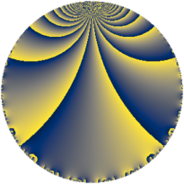# Properties

 Label 144.3.e.bLevel $144$ Weight $3$ Character orbit 144.e Analytic conductor $3.924$ Analytic rank $0$ Dimension $2$ CM no Inner twists $2$

# Related objects

## Newspace parameters

 Level: $$N$$ $$=$$ $$144 = 2^{4} \cdot 3^{2}$$ Weight: $$k$$ $$=$$ $$3$$ Character orbit: $$[\chi]$$ $$=$$ 144.e (of order $$2$$, degree $$1$$, not minimal)

## Newform invariants

 Self dual: no Analytic conductor: $$3.92371580679$$ Analytic rank: $$0$$ Dimension: $$2$$ Coefficient field: $$\Q(\sqrt{-2})$$ Defining polynomial: $$x^{2} + 2$$ Coefficient ring: $$\Z[a_1, \ldots, a_{5}]$$ Coefficient ring index: $$3$$ Twist minimal: no (minimal twist has level 18) Sato-Tate group: $\mathrm{SU}(2)[C_{2}]$

## $q$-expansion

Coefficients of the $$q$$-expansion are expressed in terms of $$\beta = 3\sqrt{-2}$$. We also show the integral $$q$$-expansion of the trace form.

 $$f(q)$$ $$=$$ $$q + \beta q^{5} + 4 q^{7} +O(q^{10})$$ $$q + \beta q^{5} + 4 q^{7} + 4 \beta q^{11} + 8 q^{13} + 3 \beta q^{17} + 16 q^{19} -4 \beta q^{23} + 7 q^{25} -\beta q^{29} -44 q^{31} + 4 \beta q^{35} -34 q^{37} -11 \beta q^{41} + 40 q^{43} -20 \beta q^{47} -33 q^{49} -9 \beta q^{53} -72 q^{55} + 8 \beta q^{59} + 50 q^{61} + 8 \beta q^{65} -8 q^{67} -12 \beta q^{71} -16 q^{73} + 16 \beta q^{77} + 76 q^{79} + 28 \beta q^{83} -54 q^{85} -3 \beta q^{89} + 32 q^{91} + 16 \beta q^{95} + 176 q^{97} +O(q^{100})$$ $$\operatorname{Tr}(f)(q)$$ $$=$$ $$2q + 8q^{7} + O(q^{10})$$ $$2q + 8q^{7} + 16q^{13} + 32q^{19} + 14q^{25} - 88q^{31} - 68q^{37} + 80q^{43} - 66q^{49} - 144q^{55} + 100q^{61} - 16q^{67} - 32q^{73} + 152q^{79} - 108q^{85} + 64q^{91} + 352q^{97} + O(q^{100})$$

## Character values

We give the values of $$\chi$$ on generators for $$\left(\mathbb{Z}/144\mathbb{Z}\right)^\times$$.

 $$n$$ $$37$$ $$65$$ $$127$$ $$\chi(n)$$ $$1$$ $$-1$$ $$1$$

## Embeddings

For each embedding $$\iota_m$$ of the coefficient field, the values $$\iota_m(a_n)$$ are shown below.

For more information on an embedded modular form you can click on its label.

Label $$\iota_m(\nu)$$ $$a_{2}$$ $$a_{3}$$ $$a_{4}$$ $$a_{5}$$ $$a_{6}$$ $$a_{7}$$ $$a_{8}$$ $$a_{9}$$ $$a_{10}$$
17.1
 − 1.41421i 1.41421i
0 0 0 4.24264i 0 4.00000 0 0 0
17.2 0 0 0 4.24264i 0 4.00000 0 0 0
 $$n$$: e.g. 2-40 or 990-1000 Significant digits: Format: Complex embeddings Normalized embeddings Satake parameters Satake angles

## Inner twists

Char Parity Ord Mult Type
1.a even 1 1 trivial
3.b odd 2 1 inner

## Twists

By twisting character orbit
Char Parity Ord Mult Type Twist Min Dim
1.a even 1 1 trivial 144.3.e.b 2
3.b odd 2 1 inner 144.3.e.b 2
4.b odd 2 1 18.3.b.a 2
5.b even 2 1 3600.3.l.d 2
5.c odd 4 2 3600.3.c.b 4
8.b even 2 1 576.3.e.f 2
8.d odd 2 1 576.3.e.c 2
9.c even 3 2 1296.3.q.f 4
9.d odd 6 2 1296.3.q.f 4
12.b even 2 1 18.3.b.a 2
15.d odd 2 1 3600.3.l.d 2
15.e even 4 2 3600.3.c.b 4
16.e even 4 2 2304.3.h.c 4
16.f odd 4 2 2304.3.h.f 4
20.d odd 2 1 450.3.d.f 2
20.e even 4 2 450.3.b.b 4
24.f even 2 1 576.3.e.c 2
24.h odd 2 1 576.3.e.f 2
28.d even 2 1 882.3.b.a 2
28.f even 6 2 882.3.s.d 4
28.g odd 6 2 882.3.s.b 4
36.f odd 6 2 162.3.d.b 4
36.h even 6 2 162.3.d.b 4
44.c even 2 1 2178.3.c.d 2
48.i odd 4 2 2304.3.h.c 4
48.k even 4 2 2304.3.h.f 4
52.b odd 2 1 3042.3.c.e 2
52.f even 4 2 3042.3.d.a 4
60.h even 2 1 450.3.d.f 2
60.l odd 4 2 450.3.b.b 4
84.h odd 2 1 882.3.b.a 2
84.j odd 6 2 882.3.s.d 4
84.n even 6 2 882.3.s.b 4
132.d odd 2 1 2178.3.c.d 2
156.h even 2 1 3042.3.c.e 2
156.l odd 4 2 3042.3.d.a 4

By twisted newform orbit
Twist Min Dim Char Parity Ord Mult Type
18.3.b.a 2 4.b odd 2 1
18.3.b.a 2 12.b even 2 1
144.3.e.b 2 1.a even 1 1 trivial
144.3.e.b 2 3.b odd 2 1 inner
162.3.d.b 4 36.f odd 6 2
162.3.d.b 4 36.h even 6 2
450.3.b.b 4 20.e even 4 2
450.3.b.b 4 60.l odd 4 2
450.3.d.f 2 20.d odd 2 1
450.3.d.f 2 60.h even 2 1
576.3.e.c 2 8.d odd 2 1
576.3.e.c 2 24.f even 2 1
576.3.e.f 2 8.b even 2 1
576.3.e.f 2 24.h odd 2 1
882.3.b.a 2 28.d even 2 1
882.3.b.a 2 84.h odd 2 1
882.3.s.b 4 28.g odd 6 2
882.3.s.b 4 84.n even 6 2
882.3.s.d 4 28.f even 6 2
882.3.s.d 4 84.j odd 6 2
1296.3.q.f 4 9.c even 3 2
1296.3.q.f 4 9.d odd 6 2
2178.3.c.d 2 44.c even 2 1
2178.3.c.d 2 132.d odd 2 1
2304.3.h.c 4 16.e even 4 2
2304.3.h.c 4 48.i odd 4 2
2304.3.h.f 4 16.f odd 4 2
2304.3.h.f 4 48.k even 4 2
3042.3.c.e 2 52.b odd 2 1
3042.3.c.e 2 156.h even 2 1
3042.3.d.a 4 52.f even 4 2
3042.3.d.a 4 156.l odd 4 2
3600.3.c.b 4 5.c odd 4 2
3600.3.c.b 4 15.e even 4 2
3600.3.l.d 2 5.b even 2 1
3600.3.l.d 2 15.d odd 2 1

## Hecke kernels

This newform subspace can be constructed as the kernel of the linear operator $$T_{5}^{2} + 18$$ acting on $$S_{3}^{\mathrm{new}}(144, [\chi])$$.

## Hecke characteristic polynomials

$p$ $F_p(T)$
$2$ $$T^{2}$$
$3$ $$T^{2}$$
$5$ $$18 + T^{2}$$
$7$ $$( -4 + T )^{2}$$
$11$ $$288 + T^{2}$$
$13$ $$( -8 + T )^{2}$$
$17$ $$162 + T^{2}$$
$19$ $$( -16 + T )^{2}$$
$23$ $$288 + T^{2}$$
$29$ $$18 + T^{2}$$
$31$ $$( 44 + T )^{2}$$
$37$ $$( 34 + T )^{2}$$
$41$ $$2178 + T^{2}$$
$43$ $$( -40 + T )^{2}$$
$47$ $$7200 + T^{2}$$
$53$ $$1458 + T^{2}$$
$59$ $$1152 + T^{2}$$
$61$ $$( -50 + T )^{2}$$
$67$ $$( 8 + T )^{2}$$
$71$ $$2592 + T^{2}$$
$73$ $$( 16 + T )^{2}$$
$79$ $$( -76 + T )^{2}$$
$83$ $$14112 + T^{2}$$
$89$ $$162 + T^{2}$$
$97$ $$( -176 + T )^{2}$$